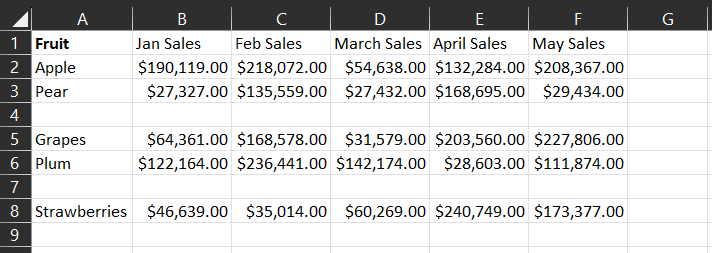In my line of work as a scientist, I often find myself working with various sets of data. This data could range from monitoring office comfort and air quality to analyzing test results for different samples, like water samples. To better understand and present this information, I frequently turn to Microsoft Excel—a versatile tool that helps organize and visualize data effectively.

One common challenge I encounter is filtering out specific information from a column to use in various calculations. Let me share a situation you might find relatable, even if you're not deeply familiar with Excel.

Imagine you're dealing with a spreadsheet that lists fruits in one column (let's call it Column A), and the corresponding sales quantities for each month in subsequent columns (like Column B for January, Column C for February, and so on).

In this scenario, you might want to create a unique list of the different fruits in Column A. This is super easy and just involves using the UNIQUE formula which is as per the below:

``=UNIQUE(array,[by_column],[exactly_once])``

In the above formula, the array is the range you want to look at the entries in, for example in mine I would look at A1:A8, by columns is how to compare, do we compare by row (which is default or FALSE) or by column (if TRUE), and exactly once is that we look for entries that occur once (if true), or if false, all unique values (which is default). By column and Exactly once are not required, so we could look at =UNIQUE(A1:A8) as our formula.

However, there's a little wrinkle. Sometimes, due to formatting issues or missing data, you end up with empty cells (also represented as “0”) in the list. These empty cells can complicate your analysis.

Here's where the FILTER formula comes in. This formula is like a smart filter for your data. It helps you extract specific records based on certain criteria. The formula's structure is as follows:

``=FILTER(array, criteria, [range_if_empty])``

Let me break it down. “Array” refers to the range or collection of data you want to filter. “Criteria” is the rule you set for the filter to determine what to include. The optional “range_if_empty” parameter lets you specify what to show if no data meets the criteria.

Let's circle back to our fruit scenario. To eliminate those pesky empty cells, you can use the FILTER formula. Here's how it works:

``=FILTER(Sheet1!A1:A11, Sheet1!A1:A11<>"")``

In plain terms, this formula scans the range (Sheet1!A1:A11) and only selects entries that are not blank (indicated by <> “”). This leaves you with a list of fruits without those troublesome empty cells. However, this isn't about getting unique entries just yet—there might be duplicates of the same fruit (I added duplicates in a new set of data to demonstrate this).

To trim down to unique entries, we can add another layer using the UNIQUE formula within the FILTER formula. Here's how:

``=UNIQUE(FILTER(Sheet1!A1:A11, Sheet1!A1:A11<>""))``

With this enhanced formula, you're filtering out the blanks and then distilling the list to show only the unique fruits. This technique is super handy whether you're excluding data from specific columns or narrowing down your dataset by other criteria, like regions or any other specifics you need.

So, there you have it—a powerful tool called FILTER that can significantly refine your data processing. Next time you're working with Excel, remember this technique to make your data analysis smoother and more insightful.